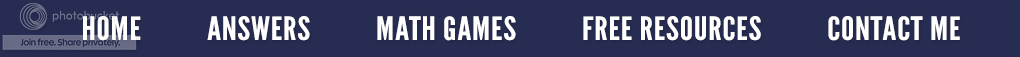### The Division House Mystery

When writing division problems, we can use three different forms, a fraction, the division symbol (÷) or the division house. Why it has been called the division "house" has always been a mystery to me since most math symbols are named something that sounds important. I tried looking it up on-line, but never found a formal mathematical word. Well the mystery has been partially solved thanks to SamizdatMath on Teachers Pay Teachers who mentioned the word vinculum.
I decided to research "vinculum", and here is what I discovered. It is a Latin word that means to ‘bond’ or ‘tie’, and was first used by Michael Stifel in 1544 in Arithmetica integra. It is the horizontal line used to separate the numerator and denominator in a fraction. We also see it above the digital pattern that repeats in a repeating decimal or in geometry above two letters that represent a line segment.

Originally the line was placed under the items to be grouped. What today might be written 7(3x + 4) the early users of the vinculum would write 3x + 4. Today that line is placed over the items to be grouped. The line of a radical sign or the long division house is also called a vinculum.

The symbol is utilized to separate the dividend from the divisor, and is drawn as a right parenthesis with an attached vinculum (see illustration above) extending to the right. The vinculum shows that the digits of the dividend are to be kept together as they represent one whole number.

But when it is all said and done, the entire division "house" symbol seems to have no established name of its own. How mathematically sad! Consequently, it has simply be termed the "long division symbol," or sometimes the division "bracket" or division "house". So the next time you draw the symbol on the board, impress your students with the math word "vinculum"!Can you find the vinculums in this cartoon?

### It's A Square Deal

My math-a-magical powers are back.  In my January 23rd post, I demonstrated how to easily divide by the decimal .25.  Today let's look put on our magician's hat and learn how to square any two digit number that ends in 5.

In the number below, look at the digit in the tens place (3) and count up one more.  In this case when we count up, the 3 becomes a 4.  Now multiply the two numbers together, 4 × 3 which equals 12.  Next do 52 which is 5 × 5 = 25.  Therefore, the answer to 352 is 1,225.

Let's try another one.  Remember, it must be a two digit number that ends in 5 for the magic trick to work. This time let's start with 65 and follow the exact same procedure used above.

Did you get the right answer?  Have your students try this using all the two digits numbers that end in 5, beginning with 15 and finishing with 95.  Since all math is based on patterns, ask hem to carefully look for a pattern and describe it.  I think you will be very surprised at what you find!